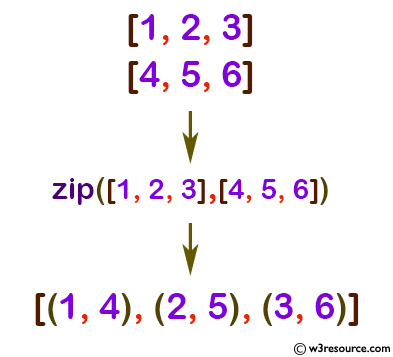# Python: zip() function

## zip() function

The zip() function is used to make an iterator that aggregates elements from each of the iterables. The iterator stops when the shortest input iterable is exhausted. With a single iterable argument, it returns an iterator of 1-tuples. With no arguments, it returns an empty iterator.

Version:

(Python 3.2.5)

Syntax:

```zip(*iterables)
```

Parameter:

Name Description Required /
Optional
*iterables Iterator objects that will be joined together. Required

Return value:

Returns an iterator of tuples, where the i-th tuple contains the i-th element from each of the argument sequences or iterables.

Example: Python zip() function

``````x = [1, 2, 3]
y = [4, 5, 6]
zipped = zip(x, y)
print(list(zipped))
``````

Output:

```[(1, 4), (2, 5), (3, 6)]
```

Pictorial Presentation:Python Code Editor:

Previous: vars()
Next: Python Home

Test your Python skills with w3resource's quiz

﻿

## Python: Tips of the Day

How do I check if a list is empty?

For example, if passed the following:

```a = []
if not a:
print("List is empty")
```

Ref: https://bit.ly/2A4JXx9